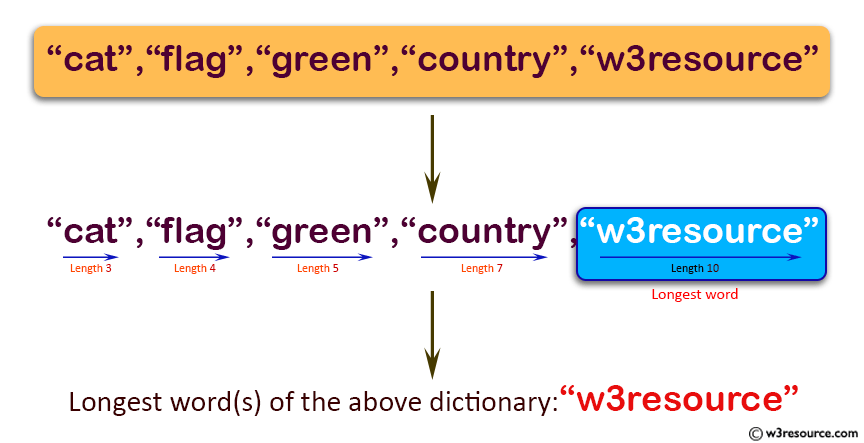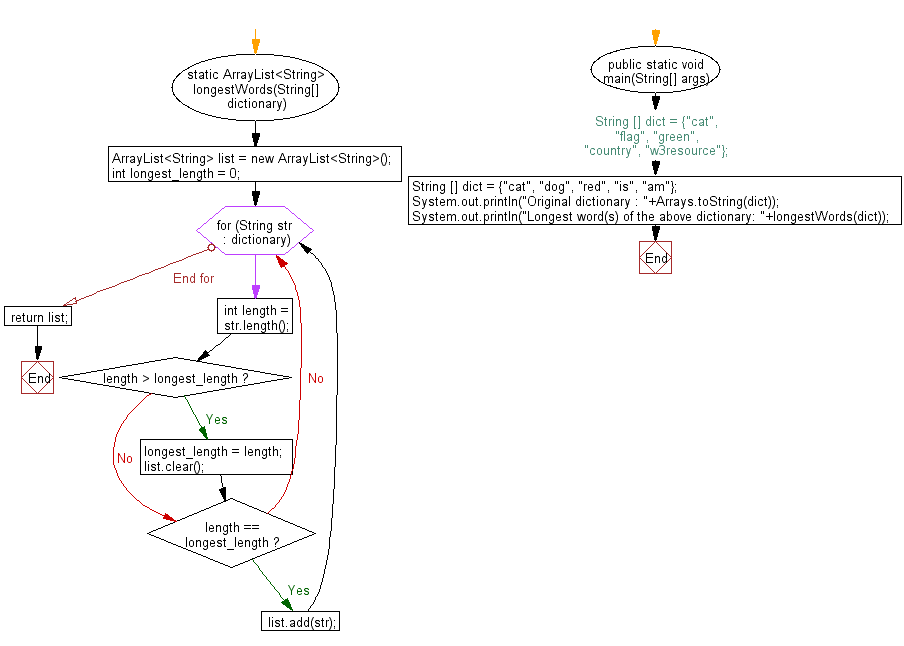﻿ Java: Find all of the longest word in a given dictionary# Java Exercises: Find all of the longest word in a given dictionary

## Java Basic: Exercise-138 with Solution

Write a Java program to find all of the longest word in a given dictionary.

Example-1:
{
"cat",
"flag",
"green",
"country",
"w3resource"
}
Result: "w3resource"
Example-1:
{
"cat",
"dog",
"red",
"is",
"am"
}
Result: "cat", "dog", "red"

Pictorial Presentation:Sample Solution:

Java Code:

``````import java.util.*;
public class Solution {
static ArrayList<String> longestWords(String[] dictionary) {
ArrayList<String> list = new ArrayList<String>();
int longest_length = 0;
for (String str : dictionary) {
int length = str.length();
if (length > longest_length) {
longest_length = length;
list.clear();
}
if (length == longest_length) {
}
}
return list;
}

public static void main(String[] args) {
//String [] dict = {"cat", "flag", "green", "country", "w3resource"};
String [] dict = {"cat", "dog", "red", "is", "am"};
System.out.println("Original dictionary : "+Arrays.toString(dict));
System.out.println("Longest word(s) of the above dictionary: "+longestWords(dict));
}
}
```
```

Sample Output:

```Original dictionary : [cat, dog, red, is, am]
Longest word(s) of the above dictionary: [cat, dog, red]
```

Flowchart:Java Code Editor:

What is the difficulty level of this exercise?

Test your Programming skills with w3resource's quiz.

﻿

## Java: Tips of the Day

countOccurrences

Counts the occurrences of a value in an array.

Use Arrays.stream().filter().count() to count total number of values that equals the specified value.

```public static long countOccurrences(int[] numbers, int value) {
return Arrays.stream(numbers)
.filter(number -> number == value)
.count();
}
```

Ref: https://bit.ly/3kCAgLb